# Useful for changing permissions on Linux! How to count up to 31 with one hand.

## What to do this time

I will explain how to count up to 31 with one hand using binary numbers. Please remember it as it will help you change file permissions on Linux.

## What is a binary number?

A binary number is a number whose digits are incremented by 2. What we usually use is a decimal number because it is carried by 10. Since it is a very difficult explanation for me, I have prepared a correspondence table between binary numbers and decimal numbers below. In binary, the number of digits increases when the number becomes 2. Similarly, the number of digits increases when the decimal number becomes 10.

Binary number Decimal number
0 0
1 1
10 2
11 3
100 4
101 5
110 6
111 7
1000 8
1001 9
1010 10

## How to count binary numbers with your finger

First, put your fist in a goo state. Then all your fingers will be folded. From this state, count the binary numbers as follows. Folded fingers = 0 Standing finger = 1

In this state, it is 0 in decimal, so it is 0 in decimal.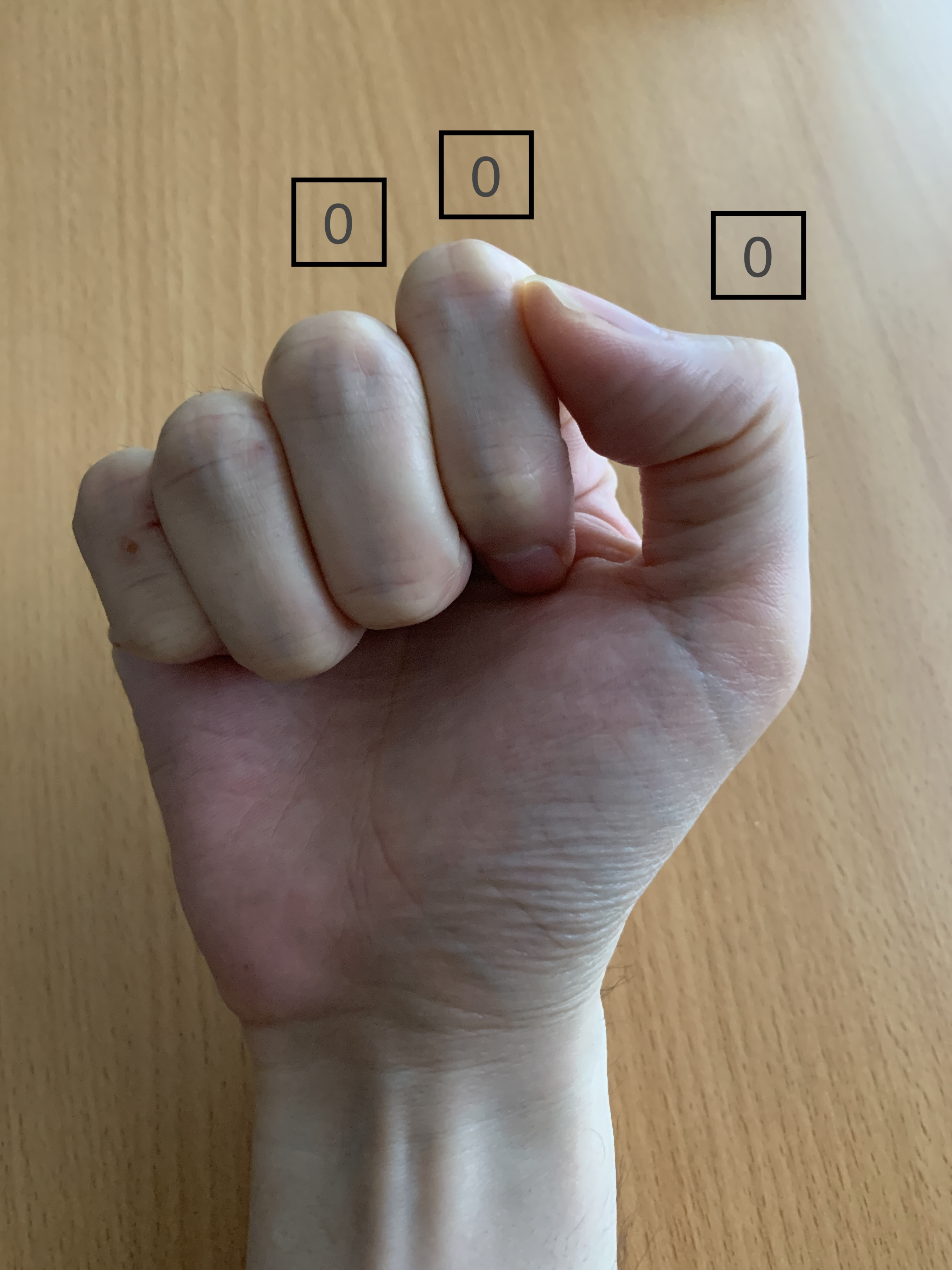1 in decimal, 1 in decimal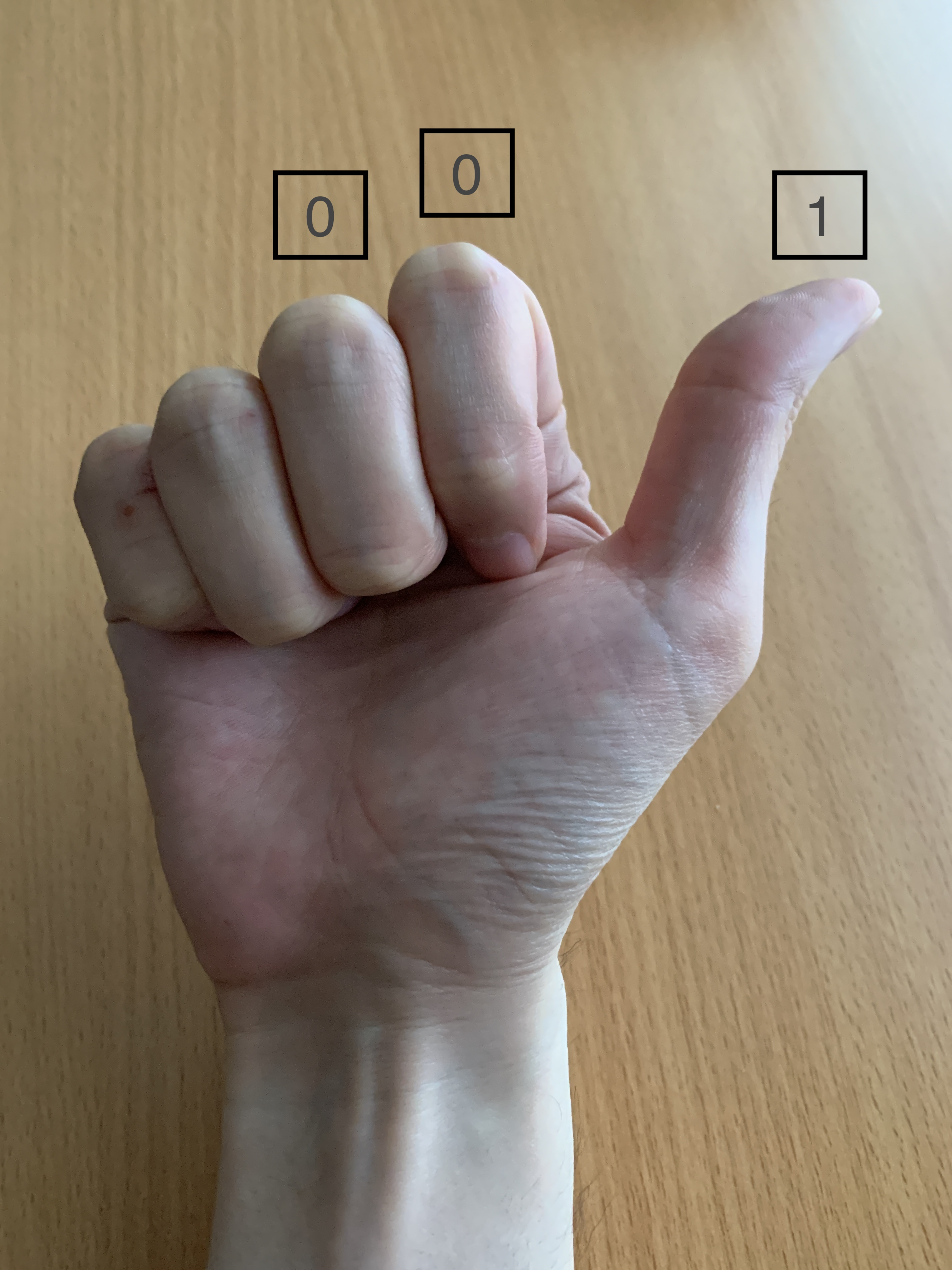10 in decimal, 2 in decimal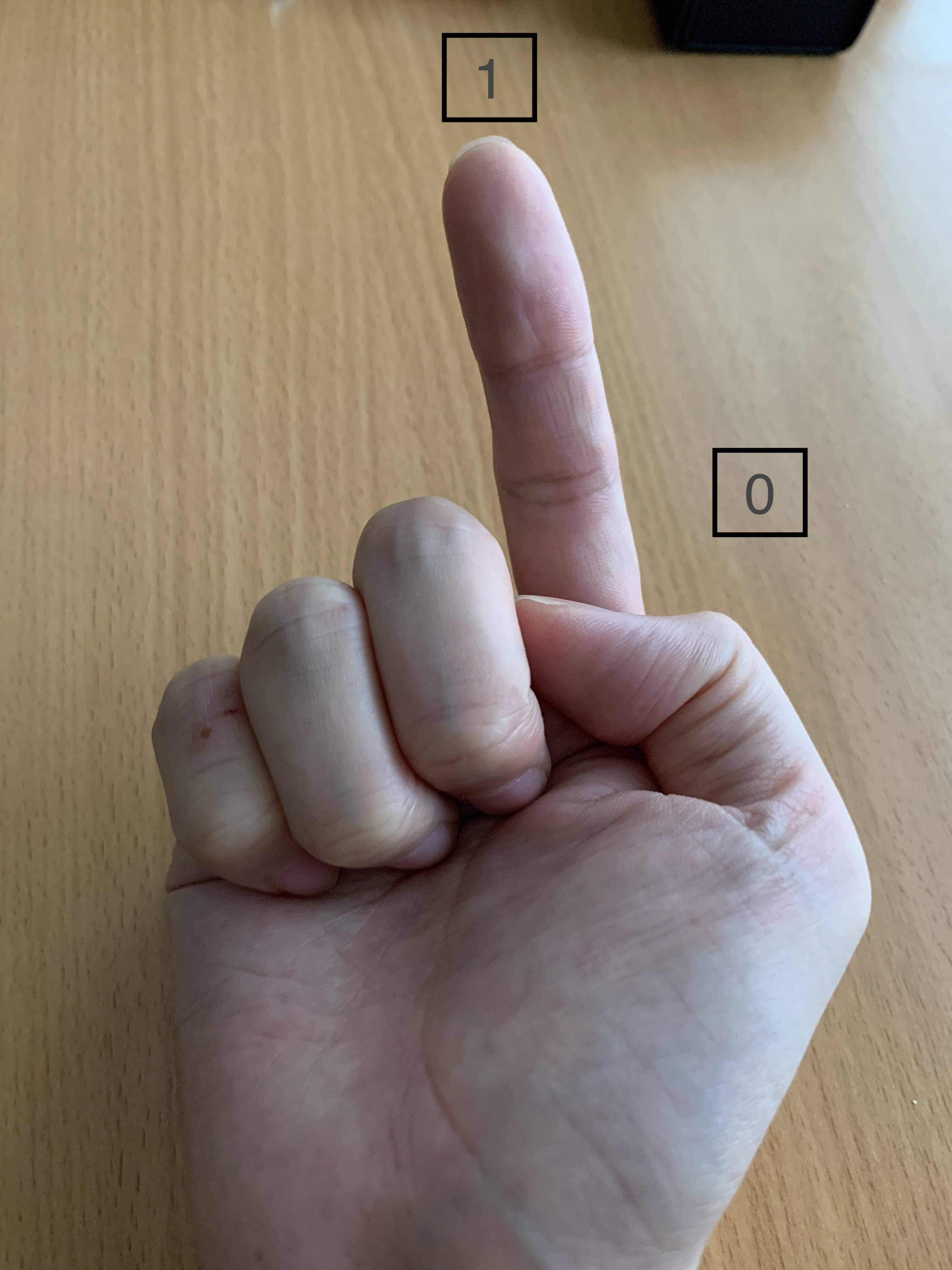11 in decimal, 3 in decimal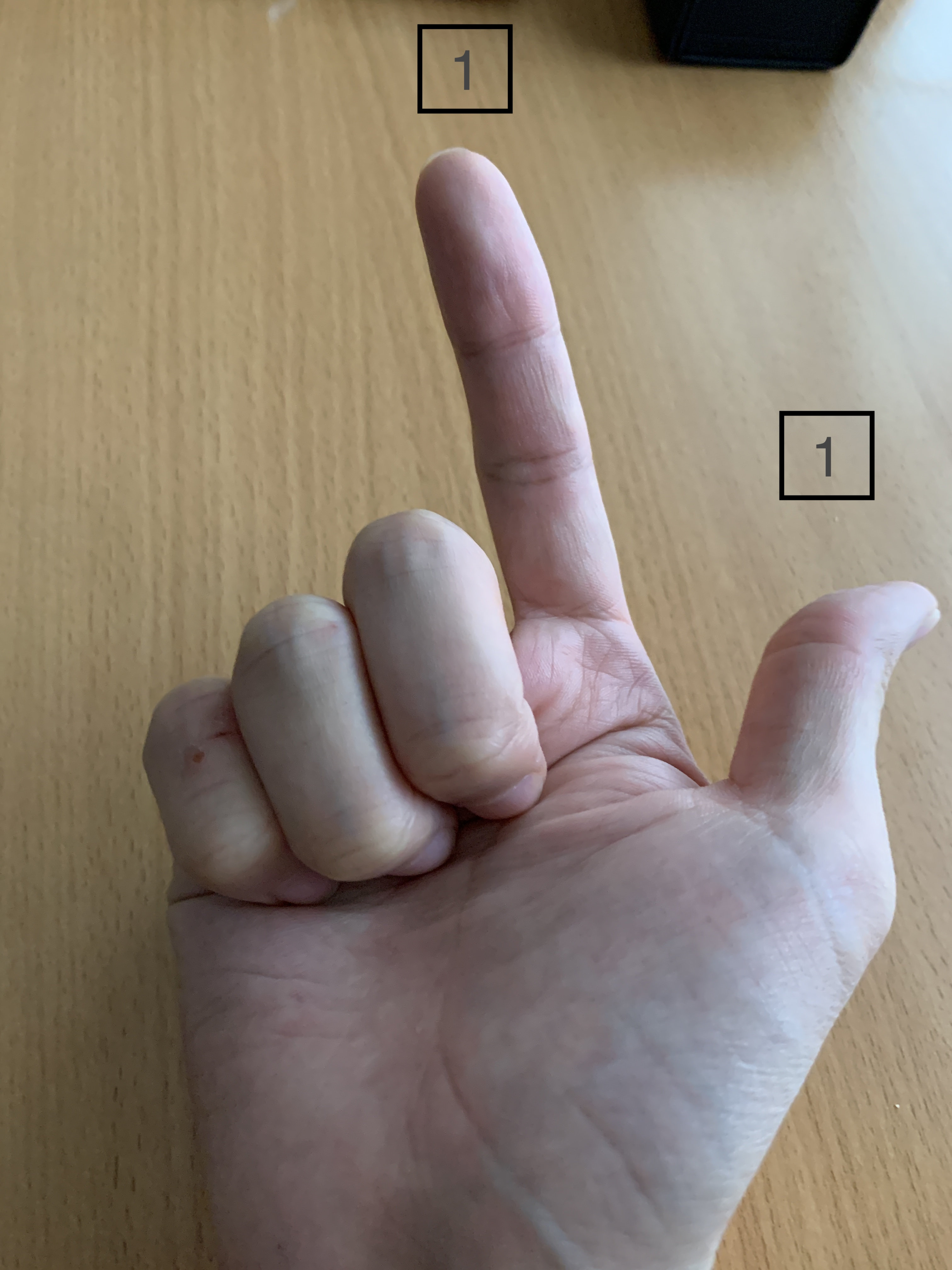100 in decimal, 4 in decimal (not f ◯ ck)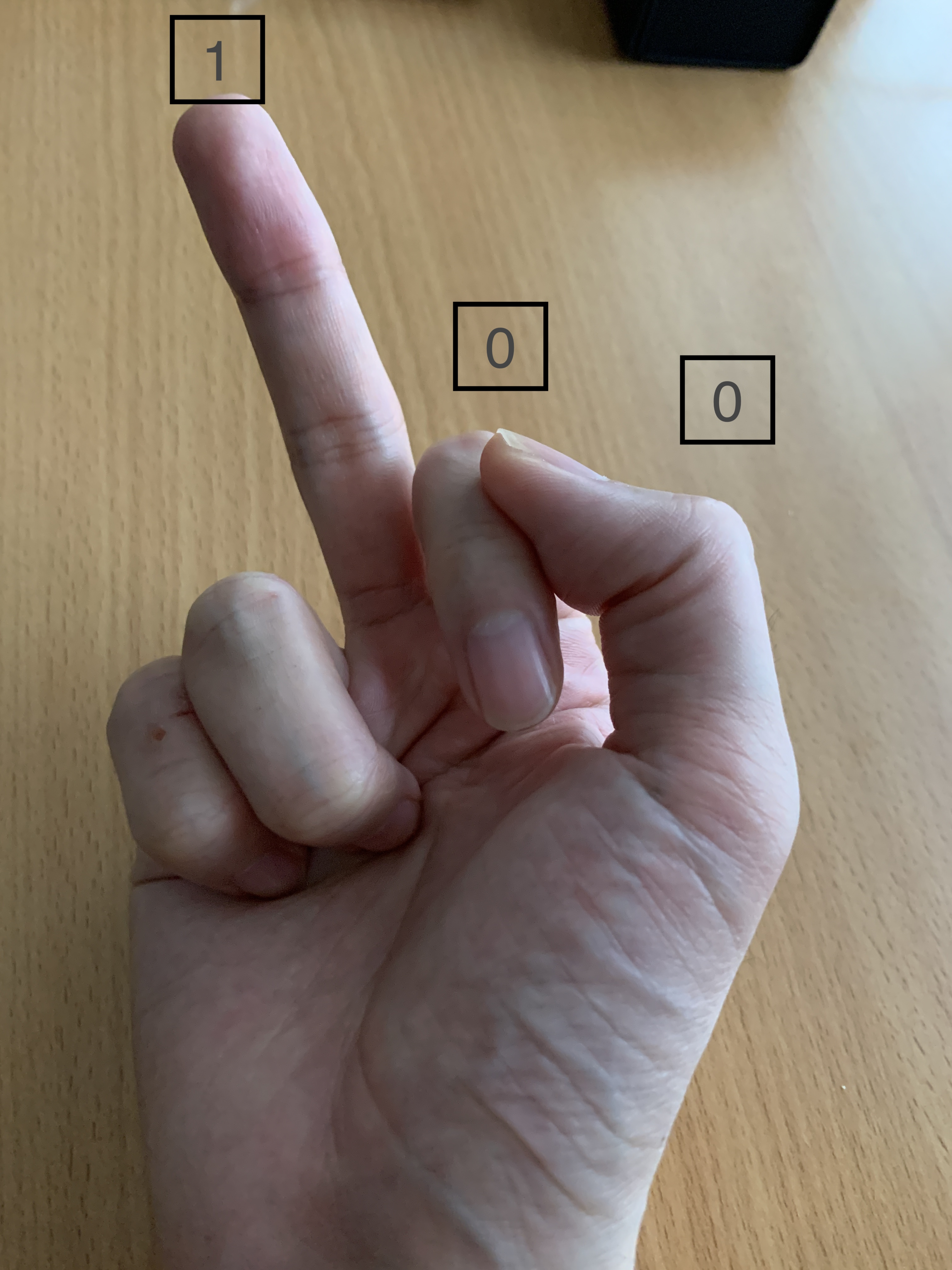101 in decimal, 5 in decimal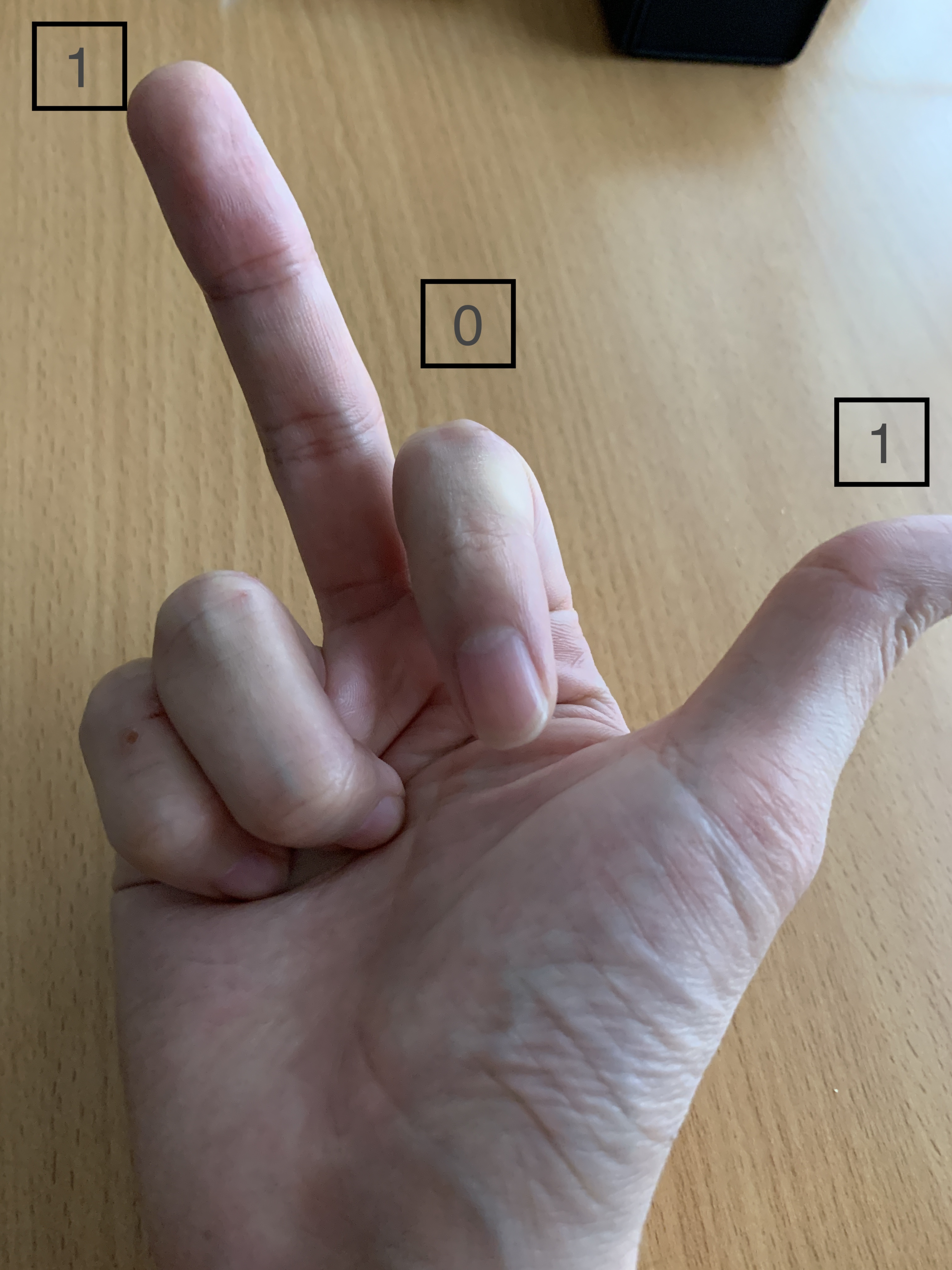110 in decimal, 6 in decimal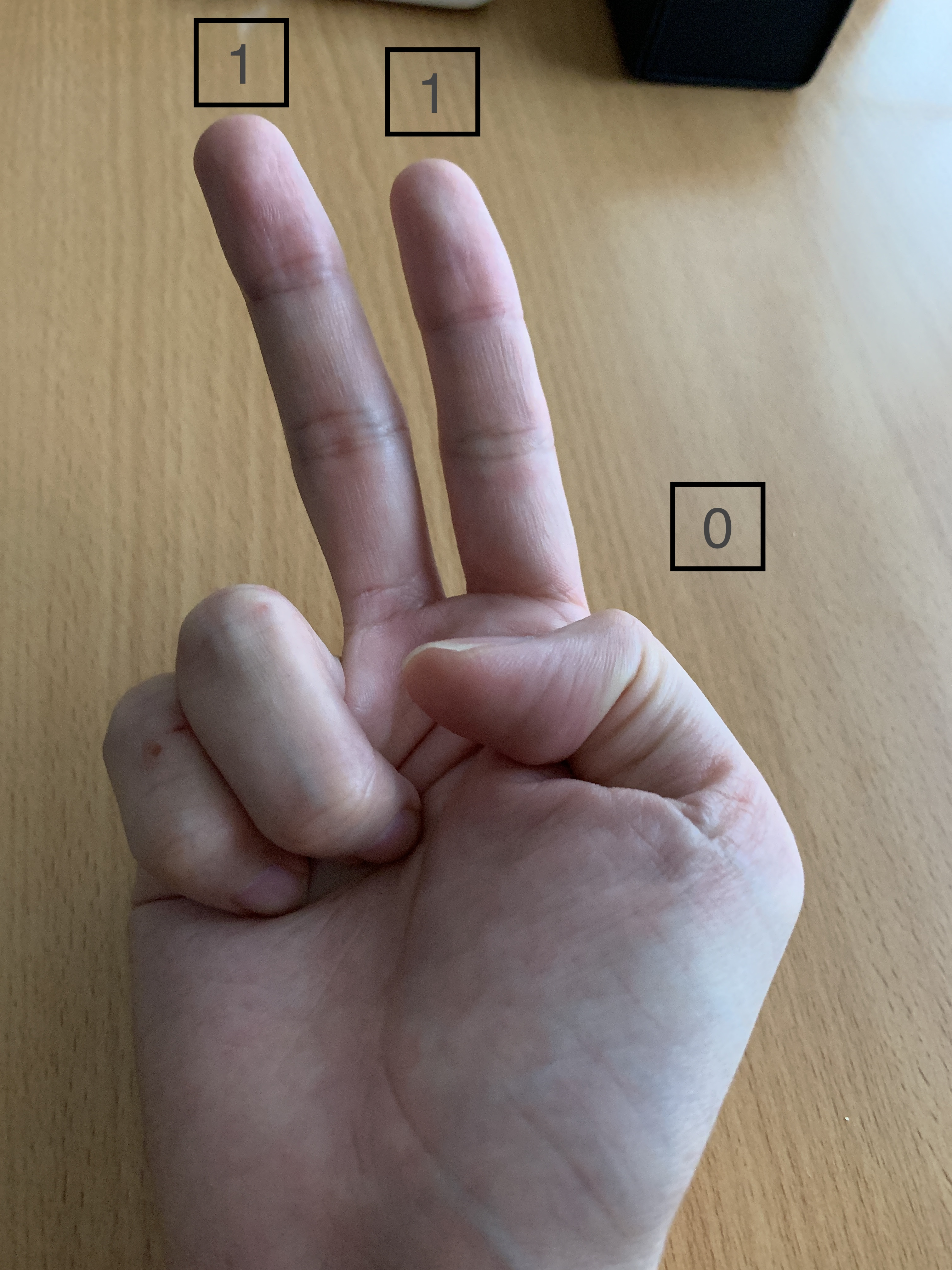111 in decimal, 7 in decimal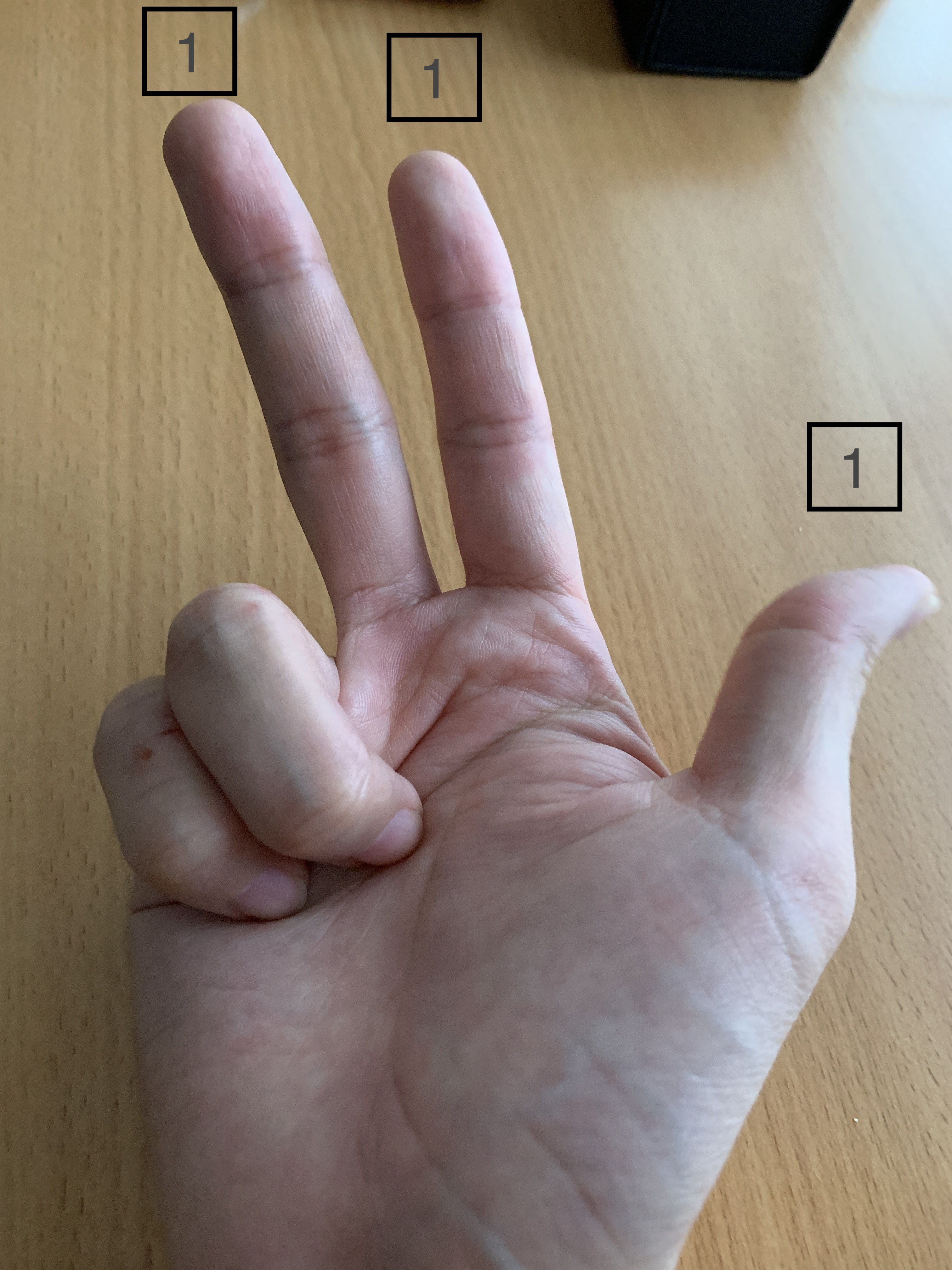You can count up to 2 to the nth power-1 (n is the number of fingers), so if you use the fingers of both hands, you can count up to 1023 with 2 to the 10th power-1. (It's not practical at all ...)

I prepared a test file on the linux server. There is a description -rwxr--r-- at the left end of the execution result of the ls command. This represents permissions and has the following implications: r = reference authority w = File edit permission x = execute permission for the file

2nd to 4th columns of permissions = What the root user can do 5th to 7th lines of permissions = What the owner of the file can do 8th to 10th lines of permission = What users who belong to the specified group can do

That is, in the example below Root user can be referenced, edited, and executed. In other words, you can do anything. You can only refer to the owner of the file Only users who belong to the group can refer to It means that.

``````# ls -l
-rwxr--r--.1 yoshida users 0 May 6 03:35 test.txt
``````

## Practice

Now let's change the permissions. This time I would like to give the owner of the file edit permission. In conclusion, you can change the permissions with the following command. The important part here is "764". This represents permissions. The first digit, 7 is 111 in binary, so you can give the root user rwx privileges. (That is, no change) The second digit, 6 is 110 in binary, so you can give the owner rw privileges. The second digit, 4 is 100 in binary, so you can give the owner r privileges. (That is, no change)

``````# chmod 764 test.txt
``````
``````# ls -l
-rwxrw-r--.1 yoshida users 0 May 6 03:35 test.txt
``````

The flow in my head is shown below.

1. Edit the 5th to 7th digits because the permission of the file owner is changed.
2. Since edit authority is given, change from "r--" to "rw-"
3. "rw-" means 110 in decimal (move your finger here to count how many 110 is in decimal) 4.2 The decimal number 110 is 6! Therefore, it is possible to give the owner edit permission with "chmod 764".

## At the end

That is all for this article. It's easy to do, so try it on AWS EC2, or try launching a Linux server by referring to the article I wrote earlier! ·reference I made a virtual server with VMware vSphere I installed CentOS on a virtual machine